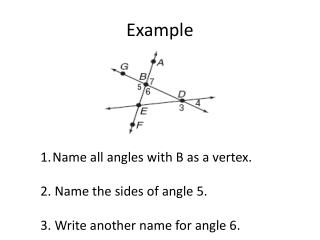DownloadDownload PresentationExample

# Example

Download Presentation## Example

- - - - - - - - - - - - - - - - - - - - - - - - - - - E N D - - - - - - - - - - - - - - - - - - - - - - - - - - -
##### Presentation Transcript

1. Example Name all angles with B as a vertex. 2. Name the sides of angle 5. 3. Write another name for angle 6.

2. Example Classify each angle as right, obtuse, acute or straight. 1. Angle TYV 2. Angle WYT 3. Angle TYU 4. angleTYX

3. Angle Relationship Sec 1.5 Sol: G.3 and G.11

4. Adjacent Angles Definition: 2 angles that lie on the same plane, have a common vertex and a common side, but have no common interior points. Example: Non – Example: A D C C B D B A No Common Vertex

5. Vertical Angles Definition: 2 non-adjacent angles formed by two intersecting lines. A pair of angles whose sides form opposite rays. Examples: Lesson 1-5: Pairs of Angles

6. Vertical Angles Cont. Non – Example: Remember: To form a vertical pair you have to have two intersection lines.

7. Linear Pair Definition: Is a pair of adjacent angles who’s non-common sides are opposite rays. Example: Non-example:

8. EX 1: Identify angle pairs 2 3 1 4 5 Identify the vertical pairs. Identify all the linear pairs. Identify the Adjacent angles.

9. Complementary Angles Definition: A pair of angles whose sum is 90˚ Examples: Even when two angles are non adjacent they can be Added together to form a 90 degree angles Lesson 1-5: Pairs of Angles

10. Example 3- The measure of two complimentary angles are 16z-9 and 4z+3. Find the measures of the two angles.

11. Supplementary Angles Definition: A pair of angles whose sum is 180˚ Examples: Adjacent supplementary angles are also called “Linear Pair.” Non-Adjacent Angles Lesson 1-5: Pairs of Angles

12. Example 4Find the value of x and the measure of each angle (2x+6)° (3x-1)°

13. Perpendicular Lines Definitions: Lines that form two right angles. Symbolized By :

14. Example 6 X Y Z W